# Monacor

Monacor develops and produces products for HiFi, Car HiFi, PA, Multimedia und Home Cinema. nacor

## Full Range## Monacor SP-15

order no. mo-100310

EUR 32,70excl. VAT: € 27.48 / \$ 29.68

.

• power handling (continuous/programme) = 10/15 W
• frequency range = 430-8000 Hz
• resonance frequency fs = 430 Hz
• impedance R = 8 Ohm
• sound pressure level SPL = 96 dB (2,83V; 1m)
• mounting diameter d = 79 mm
• overall diameter d = 85x39 mm
• mounting depth (not countersunk) t = 39 mm
• mass m = 0,355 kg
PDF data sheet

## Monacor MS-55

order no. mo-100260

EUR 16,96excl. VAT: € 14.25 / \$ 15.39

.

• power handling (continuous/programme) = W
• frequency range = 200-20000 Hz
• resonance frequency fs = 200 Hz
• impedance R = 8 Ohm
• sound pressure level SPL = 85 dB (2,83V; 1m)
• mounting diameter d = 50 mm
• overall diameter d = 74x55 mm
• mounting depth (not countersunk) t = 30 mm
• mass m = 0,21 kg
PDF data sheet## Monacor SP-5/4

order no. mo-103500

EUR 9,37excl. VAT: € 7.87 / \$ 8.50

4 cm full range driver with high quality cone.

• power handling (continuous/programme) = 2/4 W
• impedance R = 4 Ohm
• mounting diameter d = 47 mm
• overall diameter d = 50 mm
• mounting depth (not countersunk) t = 21 mm## Monacor SP-5/8

order no. mo-104570

EUR 10,92excl. VAT: € 9.18 / \$ 9.91

4 cm full range driver with high quality cone.

• power handling (continuous/programme) = 6/12 W
• impedance R = 8 Ohm
• mounting diameter d = 47 mm
• overall diameter d = 50 mm
• mounting depth (not countersunk) t = 24 mm## Monacor SP-6/4

order no. mo-103520

EUR 5,76excl. VAT: € 4.84 / \$ 5.23

5 cm full range driver with high quality cone.

• power handling (continuous/programme) = 3/5 W
• impedance R = 4 Ohm
• mounting diameter d = 52,5 mm
• overall diameter d = 57 mm
• mounting depth (not countersunk) t = 25,5 mmopen a bigger photo

recommended cabinet 1:
closed cabinet with 0,06 L volume
from 301/190 Hz (-3dB/-8dB)

recommended cabinet 2:
0,24 L volume bass reflex cabinet
with 2cm-Rohr reflex tube, 16 cm long.
from 147/119 Hz (-3dB/-8dB).

## Monacor SPX-20M

order no. mo-100930

EUR 67,90excl. VAT: € 57.06 / \$ 61.62

5 cm full range driver with high quality cone. The magnet system is based on Neodymium technology.

• power handling (continuous/programme) = 15/30 W
• frequency range = 250-22000 Hz
• resonance frequency fs = 157 Hz
• impedance R = 8 Ohm
• sound pressure level SPL = 87 dB (2,83V; 1m)
• DC resistance Re = 6,9 Ohm
• force factor BL = 4,5 N/A
• voice coil inductance L = 0,13 mH
• effective piston radiating area Sd = 13 cm2
• effective mechanical mass incl. air load mms = 1,3 g
• equivalent volume of compliance Vas = 0,2 l
• total Q factor Qts = 0,42 (Qms=4,52, Qes=0,46)
• voice coil diameter = 25 mm
• maximum peak linear excursion vibration xlin = +/- 0,5 mm
• mounting diameter d = 51,5 mm
• overall diameter d = 57 mm
• mounting depth (not countersunk) t = 37,5 mm
• mass m = 0,17 kg## Monacor SP-8/4

order no. mo-103600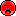- not available

8 cm full range driver with high quality cone.

• power handling (continuous/programme) = 10/20 W
• impedance R = 4 Ohm
• mounting diameter d = 70 mm
• overall diameter d = 90 mm
• mounting depth (not countersunk) t = 38 mm

## Monacor SPX-30M

order no. mo-101650

EUR 26,61excl. VAT: € 22.36 / \$ 24.15

8 cm full range driver with polypropylene cone. The driver is magnetically shielded.

• power handling (continuous/programme) = 20/40 W
• frequency range = 100-18000 Hz
• resonance frequency fs = 100 Hz
• impedance R = 8 Ohm
• sound pressure level SPL = 86 dB (2,83V; 1m)
• DC resistance Re = 6,4 Ohm
• force factor BL = 3,05 N/A
• voice coil inductance L = 0,18 mH
• effective piston radiating area Sd = 32 cm2
• effective mechanical mass incl. air load mms = 2,1 g
• equivalent volume of compliance Vas = 1,78 l
• total Q factor Qts = 0,71 (Qms=3,45, Qes=0,89)
• voice coil diameter = 20 mm
• maximum peak linear excursion vibration xlin = +/- 1,1 mm
• mounting diameter d = 71 mm
• overall diameter d = 93 mm
• mounting depth (not countersunk) t = 55 mm
• mass m = 0,5 kgopen a bigger photo

recommended cabinet 1:
closed cabinet with 1,2 L volume
from 151/95 Hz (-3dB/-8dB)

recommended cabinet 2:
4,8 L volume bass reflex cabinet
with HP35 reflex tube, 6 cm long.
from 67/59 Hz (-3dB/-8dB).

## Monacor SPX-31M

order no. mo-101660

EUR 27,66excl. VAT: € 23.24 / \$ 25.10

8 cm full range driver with paper cone. The driver is magnetically shielded.

• power handling (continuous/programme) = 20/40 W
• frequency range = 150-18000 Hz
• resonance frequency fs = 110 Hz
• impedance R = 8 Ohm
• sound pressure level SPL = 86 dB (2,83V; 1m)
• DC resistance Re = 6,4 Ohm
• force factor BL = 3,64 N/A
• voice coil inductance L = 0,18 mH
• effective piston radiating area Sd = 32 cm2
• effective mechanical mass incl. air load mms = 1,9 g
• equivalent volume of compliance Vas = 1,32 l
• total Q factor Qts = 0,58 (Qms=3,7, Qes=0,69)
• voice coil diameter = 20 mm
• maximum peak linear excursion vibration xlin = +/- 1,1 mm
• mounting diameter d = 71 mm
• overall diameter d = 93 mm
• mounting depth (not countersunk) t = 55 mm
• mass m = 0,5 kgopen a bigger photo

recommended cabinet 1:
closed cabinet with 1,3 L volume
from 156/99 Hz (-3dB/-8dB)

recommended cabinet 2:
5,2 L volume bass reflex cabinet
with HP35 reflex tube, 5 cm long.
from 70/61 Hz (-3dB/-8dB).

## Monacor SPX-32M

order no. mo-100940

EUR 32,90excl. VAT: € 27.65 / \$ 29.86

8 cm full range driver with paper cone. The driver is magnetically shielded.

• power handling (continuous/programme) = 20/40 W
• frequency range = 150-18000 Hz
• resonance frequency fs = 110 Hz
• impedance R = 8 Ohm
• sound pressure level SPL = 88 dB (2,83V; 1m)
• DC resistance Re = 6,6 Ohm
• force factor BL = 3,69 N/A
• voice coil inductance L = 0,18 mH
• effective piston radiating area Sd = 32 cm2
• effective mechanical mass incl. air load mms = 1,7 g
• equivalent volume of compliance Vas = 1,6 l
• total Q factor Qts = 0,56 (Qms=7,66, Qes=0,6)
• voice coil diameter = 20 mm
• maximum peak linear excursion vibration xlin = +/- 1,1 mm
• mounting diameter d = 71 mm
• overall diameter d = 93 mm
• mounting depth (not countersunk) t = 55 mm
• mass m = 0,52 kg## Monacor SP-272/8

order no. mo-102190- not available

12 cm full range driver with high quality cone.

• power handling (continuous/programme) = 5/7 W
• frequency range = 200-17000 Hz
• resonance frequency fs = 120 Hz
• impedance R = 8 Ohm
• sound pressure level SPL = 88 dB (2,83V; 1m)
• mounting diameter d = 108 mm
• overall diameter d = 131 mm
• mounting depth (not countersunk) t = 44 mm
• mass m = 0,32 kg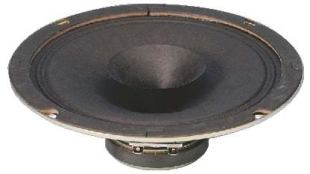## Monacor SP-276/8

order no. mo-102210

EUR 20,34excl. VAT: € 17.09 / \$ 18.46

15 cm full range driver with high quality cone.

• power handling (continuous/programme) = 5/7 W
• frequency range = 150-18000 Hz
• resonance frequency fs = 100 Hz
• impedance R = 8 Ohm
• sound pressure level SPL = 89 dB (2,83V; 1m)
• mounting diameter d = 145 mm
• overall diameter d = 165 mm
• mounting depth (not countersunk) t = 53 mm
• mass m = 0,37 kg## Monacor SP-17/4

order no. mo-103680

EUR 49,25excl. VAT: € 41.39 / \$ 44.70

16 cm full range driver with high quality cone.

• power handling (continuous/programme) = 30/60 W
• impedance R = 4 Ohm
• mounting diameter d = 147 mm
• overall diameter d = 169 mm
• mounting depth (not countersunk) t = 79 mm

## Monacor SPX-200WP

order no. mo-105560- not available

19 cm full range driver with polypropylene cone.

• power handling (continuous/programme) = 50/100 W
• frequency range = 45-18000 Hz
• resonance frequency fs = 36 Hz
• impedance R = 8 Ohm
• sound pressure level SPL = 89 dB (2,83V; 1m)
• DC resistance Re = 7,6 Ohm
• force factor BL = 6,79 N/A
• voice coil inductance L = 0,35 mH
• effective piston radiating area Sd = 213 cm2
• effective mechanical mass incl. air load mms = 19,7 g
• equivalent volume of compliance Vas = 60,5 l
• total Q factor Qts = 0,59 (Qms=4,18, Qes=0,69)
• voice coil diameter = 25 mm
• maximum peak linear excursion vibration xlin = +/- 2 mm
• mounting diameter d = 180 mm
• overall diameter d = 203 mm
• mounting depth (not countersunk) t = 89 mm
• mass m = 1,4 kg

## Monacor SPM-200X/4

order no. mo-105370

EUR 35,86excl. VAT: € 30.13 / \$ 32.55

19 cm full range driver with paper cone.

• power handling (continuous/programme) = 30/60 W
• frequency range = 100-15000 Hz
• resonance frequency fs = 89 Hz
• impedance R = 4 Ohm
• sound pressure level SPL = 94 dB (2,83V; 1m)
• DC resistance Re = 3,7 Ohm
• force factor BL = 3,9 N/A
• voice coil inductance L = 0,2 mH
• effective piston radiating area Sd = 201 cm2
• effective mechanical mass incl. air load mms = 9,9 g
• equivalent volume of compliance Vas = 14,9 l
• total Q factor Qts = 0,97 (Qms=2,69, Qes=1,52)
• voice coil diameter = 25 mm
• maximum peak linear excursion vibration xlin = +/- 1 mm
• mounting diameter d = 184 mm
• overall diameter d = 205 mm
• mounting depth (not countersunk) t = 82 mm
• mass m = 0,7 kg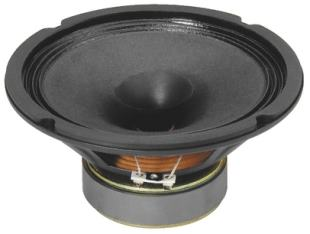recommended cabinet 1:
closed cabinet with 28 L volume
from 78/49 Hz (-3dB/-8dB)

recommended cabinet 2:
116 L volume bass reflex cabinet
with 4x HP100 reflex tube, 35 cm long.
from 36/30 Hz (-3dB/-8dB).

## Monacor SP-200 X

order no. mo-100400

EUR 99,90excl. VAT: € 83.95 / \$ 90.67

19 cm full range driver with paper cone.

• power handling (continuous/programme) = 70/35 W
• frequency range = 60-15000 Hz
• resonance frequency fs = 50 Hz
• impedance R = 8 Ohm
• sound pressure level SPL = 92 dB (2,83V; 1m)
• DC resistance Re = 7,2 Ohm
• force factor BL = 6,1 N/A
• voice coil inductance L = 0,3 mH
• effective piston radiating area Sd = 210 cm2
• effective mechanical mass incl. air load mms = 11,5 g
• equivalent volume of compliance Vas = 48 l
• total Q factor Qts = 0,52 (Qms=1,96, Qes=0,7)
• voice coil diameter = 25,5 mm
• maximum peak linear excursion vibration xlin = +/- 1,6 mm
• mounting diameter d = 182 mm
• overall diameter d = 205 mm
• mounting depth (not countersunk) t = 80 mm
• mass m = 1,3 kg## Monacor SP-205/8

order no. mo-104460

EUR 29,03excl. VAT: € 24.39 / \$ 26.35

19 cm full range driver with paper cone.

• power handling (continuous/programme) = 8/10 W
• frequency range = 300-17500 Hz
• resonance frequency fs = 100 Hz
• impedance R = 8 Ohm
• sound pressure level SPL = 94 dB (2,83V; 1m)
• mounting diameter d = 180 mm
• overall diameter d = 205 mm
• mounting depth (not countersunk) t = 63 mm
• mass m = 0,37 kg## Monacor SP-7W

order no. mo-100750

EUR 20,86excl. VAT: € 17.53 / \$ 18.93

10,2 x 10,2 cm full range driver with high quality cone.

• power handling (continuous/programme) = 4/7 W
• frequency range = 300-10000 Hz
• resonance frequency fs = 160 Hz
• impedance R = 8 Ohm
• sound pressure level SPL = 86 dB (2,83V; 1m)
• mounting diameter d = 95 mm
• overall diameter d = 102x102 mm
• mounting depth (not countersunk) t = 40 mm
• mass m = 0,3 kg## Monacor SP-40

order no. mo-100670

EUR 25,90excl. VAT: € 21.76 / \$ 23.51

10,3 x 10,3 cm full range driver with high quality cone. The rubber surround is invers.

• power handling (continuous/programme) = 8/16 W
• frequency range = 100-10000 Hz
• resonance frequency fs = 80 Hz
• impedance R = 8 Ohm
• sound pressure level SPL = 86 dB (2,83V; 1m)
• mounting diameter d = 95 mm
• overall diameter d = 103x103 mm
• mounting depth (not countersunk) t = 55 mm
• mass m = 0,7 kg## Monacor SP-10/4

order no. mo-103640- not available

10,5 x 10,5 cm full range driver with high quality cone.

• power handling (continuous/programme) = 15/25 W
• impedance R = 4 Ohm
• mounting diameter d = 103 mm
• overall diameter d = 105x105 mm
• mounting depth (not countersunk) t = 55 mm## Monacor SP-10/4S

order no. mo-103650

EUR 23,85excl. VAT: € 20.04 / \$ 21.65

10,5 x 10,5 cm full range driver with high quality cone. The driver is magnetically shielded.

• power handling (continuous/programme) = 15/25 W
• impedance R = 4 Ohm
• mounting diameter d = 103 mm
• overall diameter d = 105x105 mm
• mounting depth (not countersunk) t = 64 mm## Monacor SP-170

order no. mo-100350

EUR 17,63excl. VAT: € 14.82 / \$ 16.00

10,6 x 6,8 cm full range driver with high quality cone.

• power handling (continuous/programme) = 1/2 W
• impedance R = 8 Ohm
• overall diameter d = 106x68 mm
• mounting depth (not countersunk) t = 32 mm
• mass m = 0,07 kg## Monacor SP-13/4

order no. mo-103660

EUR 27,68excl. VAT: € 23.26 / \$ 25.12

12,8x 12,8 cm full range driver with high quality cone.

• power handling (continuous/programme) = 20/40 W
• impedance R = 4 Ohm
• mounting diameter d = 120 mm
• overall diameter d = 128x128 mm
• mounting depth (not countersunk) t = 60 mm
• mass m = 0,62 kg## Monacor SPH-60X

order no. mo-100920

EUR 60,83excl. VAT: € 51.12 / \$ 55.21

13 x 13 cm full range driver with high quality cone. To avoid compression effects the driver possesses a pole piece hole.

• power handling (continuous/programme) = 30/60 W
• frequency range = 100-20000 Hz
• resonance frequency fs = 60 Hz
• impedance R = 8 Ohm
• sound pressure level SPL = 89 dB (2,83V; 1m)
• DC resistance Re = 6,2 Ohm
• force factor BL = 5,1 N/A
• voice coil inductance L = 0,4 mH
• effective piston radiating area Sd = 80 cm2
• effective mechanical mass incl. air load mms = 5,9 g
• equivalent volume of compliance Vas = 9 l
• total Q factor Qts = 0,42 (Qms=2,17, Qes=0,53)
• voice coil diameter = 25,5 mm
• maximum peak linear excursion vibration xlin = +/- 1,3 mm
• mounting diameter d = 122 mm
• overall diameter d = 130x130 mm
• mounting depth (not countersunk) t = 71 mm
• mass m = 1,1 kg

journal Klang+Ton
03/2000; "Der erfolgreichste Cheap Trick aller Zeiten, wohl kein Breitbänder ist bei uns häufiger verkauft worden ... ungewohnt verfärbungsarm, schier unglaubliche Basswiedergabe für einen so kleinen Breitbänder.”order no. mo-101390

EUR 60,96excl. VAT: € 51.23 / \$ 55.33

13 x 13 cm full range driver with high quality cone. To avoid compression effects the driver possesses a pole piece hole.

• power handling (continuous/programme) = 30/60 W
• frequency range = 100-20000 Hz
• resonance frequency fs = 60 Hz
• impedance R = 8 Ohm
• sound pressure level SPL = 86 dB (2,83V; 1m)
• DC resistance Re = 6,5 Ohm
• force factor BL = 5,7 N/A
• voice coil inductance L = 0,5 mH
• effective piston radiating area Sd = 80 cm2
• effective mechanical mass incl. air load mms = 6,5 g
• equivalent volume of compliance Vas = 9,7 l
• total Q factor Qts = 0,42 (Qms=3,19, Qes=0,49)
• voice coil diameter = 25,5 mm
• maximum peak linear excursion vibration xlin = +/- 1 mm
• mounting diameter d = 122 mm
• overall diameter d = 130x130 mm
• mounting depth (not countersunk) t = 71 mm
• mass m = 1,1 kg## Monacor SP-155X

order no. mo-101360

EUR 53,50excl. VAT: € 44.96 / \$ 48.55

15,5 x 15,5 cm full range driver with paper cone.

• power handling (continuous/programme) = 25/50 W
• frequency range = 100-20000 Hz
• resonance frequency fs = 70 Hz
• impedance R = 8 Ohm
• sound pressure level SPL = 93 dB (2,83V; 1m)
• DC resistance Re = 6,5 Ohm
• force factor BL = 4,6 N/A
• voice coil inductance L = 0,4 mH
• effective piston radiating area Sd = 117 cm2
• effective mechanical mass incl. air load mms = 6,5 g
• equivalent volume of compliance Vas = 14 l
• total Q factor Qts = 0,67 (Qms=2,87, Qes=0,88)
• voice coil diameter = 25,5 mm
• maximum peak linear excursion vibration xlin = +/- 1 mm
• mounting diameter d = 146 mm
• overall diameter d = 155x155 mm
• mounting depth (not countersunk) t = 75 mm
• mass m = 1,25 kg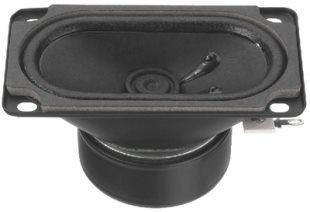## Monacor SP-59/4S

order no. mo-103700

EUR 14,13excl. VAT: € 11.87 / \$ 12.82

5 x 9 cm full range driver with high quality cone.

• power handling (continuous/programme) = 3/5 W
• impedance R = 4 Ohm
• mounting diameter d = 47x85 mm
• overall diameter d = 50x90 mm
• mounting depth (not countersunk) t = 42 mm

## Monacor SPX-21M

order no. mo-101640

EUR 28,92excl. VAT: € 24.30 / \$ 26.25

5,55 x 5,55 cm full range driver with high quality cone. The magnet system is based on Neodymium technology.

• power handling (continuous/programme) = 12/25 W
• frequency range = 250-22000 Hz
• resonance frequency fs = 170 Hz
• impedance R = 4 Ohm
• sound pressure level SPL = 84 dB (2,83V; 1m)
• DC resistance Re = 3,74 Ohm
• force factor BL = 3,32 N/A
• voice coil inductance L = 0,12 mH
• effective piston radiating area Sd = 13 cm2
• effective mechanical mass incl. air load mms = 1,45 g
• equivalent volume of compliance Vas = 0,14 l
• total Q factor Qts = 0,48 (Qms=4,66, Qes=0,53)
• voice coil diameter = 20 mm
• maximum peak linear excursion vibration xlin = +/- 1,2 mm
• mounting diameter d = 50,5 mm
• overall diameter d = 55,5x55,5 mm
• mounting depth (not countersunk) t = 34 mm
• mass m = 0,09 kg## Monacor SP-6/8SQ

order no. mo-104590

EUR 24,96excl. VAT: € 20.97 / \$ 22.65

5,5 x 5,5 cm full range driver with high quality cone.

• power handling (continuous/programme) = 10/20 W
• impedance R = 8 Ohm
• overall diameter d = 55x55 mm
• mounting depth (not countersunk) t = 34 mm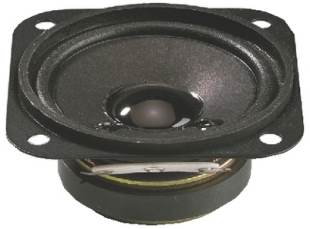## Monacor SP-6/4SQ

order no. mo-103540

EUR 9,69excl. VAT: € 8.14 / \$ 8.79

5,9 x 5,9 cm full range driver with high quality cone.

• power handling (continuous/programme) = 3/5 W
• impedance R = 4 Ohm
• mounting diameter d = 52,5 mm
• overall diameter d = 59x59 mm
• mounting depth (not countersunk) t = 26 mm## Monacor SP-6/4SQS

order no. mo-103550

EUR 6,83excl. VAT: € 5.74 / \$ 6.20

5,9 x 5,9 cm full range driver with high quality cone.

• power handling (continuous/programme) = 3/5 W
• impedance R = 4 Ohm
• mounting diameter d = 52,5 mm
• overall diameter d = 59x59 mm
• mounting depth (not countersunk) t = 33,5 mm## Monacor SP-7/4SQ

order no. mo-103580

EUR 9,56excl. VAT: € 8.03 / \$ 8.68

6,65 x 6,65 cm full range driver with high quality cone.

• power handling (continuous/programme) = 4/8 W
• impedance R = 4 Ohm
• mounting diameter d = 61 mm
• overall diameter d = 66,5x66,5 mm
• mounting depth (not countersunk) t = 26 mm## Monacor SP-7/4SQS

order no. mo-103590

EUR 11,52excl. VAT: € 9.68 / \$ 10.46

6,65 x 6,65 cm full range driver with high quality cone. The driver is magnetically shielded.

• power handling (continuous/programme) = 4/8 W
• impedance R = 4 Ohm
• mounting diameter d = 61 mm
• overall diameter d = 66,5x66,5 mm
• mounting depth (not countersunk) t = 33,5 mm## Monacor SP-30

order no. mo-101530

EUR 22,66excl. VAT: € 19.04 / \$ 20.57

7,8 x 7,8 cm full range driver with high quality cone.

• power handling (continuous/programme) = 20/50 W
• frequency range = 250-15000 Hz
• resonance frequency fs = 150 Hz
• impedance R = 4 Ohm
• sound pressure level SPL = 88 dB (2,83V; 1m)
• mounting diameter d = 73 mm
• overall diameter d = 78x78 mm
• mounting depth (not countersunk) t = 40 mm
• mass m = 0,3 kg

## Monacor SP-626/8

order no. mo-102170

EUR 12,44excl. VAT: € 10.45 / \$ 11.29

7,8 x 7,8 cm full range driver with high quality cone.

• power handling (continuous/programme) = 2/3 W
• frequency range = 300-18000 Hz
• resonance frequency fs = 200 Hz
• impedance R = 8 Ohm
• sound pressure level SPL = 87 dB (2,83V; 1m)
• mounting diameter d = 73 mm
• overall diameter d = 78x78 mm
• mounting depth (not countersunk) t = 35 mm
• mass m = 0,3 kg

## Monacor SPH-30X/4SW

order no. mo-104180

EUR 67,51excl. VAT: € 56.73 / \$ 61.27

full range driver with kevlar cone. The magnet system is based on Neodymium technology.

• power handling (continuous/programme) = 20/50 W
• frequency range = 200-17000 Hz
• resonance frequency fs = 105 Hz
• impedance R = 4 Ohm
• sound pressure level SPL = 87 dB (2,83V; 1m)
• DC resistance Re = 3,55 Ohm
• force factor BL = 3,91 N/A
• voice coil inductance L = 0,5 mH
• effective piston radiating area Sd = 30 cm2
• effective mechanical mass incl. air load mms = 2,63 g
• equivalent volume of compliance Vas = 1,06 l
• total Q factor Qts = 0,37 (Qms=9,35, Qes=0,39)
• voice coil diameter = 21 mm
• maximum peak linear excursion vibration xlin = +/- 2 mm
• mounting diameter d = 73 mm
• overall diameter d = 80,5x80,5 mm
• mounting depth (not countersunk) t = 42 mm
• mass m = 0,15 kg

## Monacor SPH-30X/8SW

order no. mo-104190

EUR 67,51excl. VAT: € 56.73 / \$ 61.27

full range driver with kevlar cone. The magnet system is based on Neodymium technology.

• power handling (continuous/programme) = 20/50 W
• frequency range = 200-17000 Hz
• resonance frequency fs = 105 Hz
• impedance R = 8 Ohm
• sound pressure level SPL = 87 dB (2,83V; 1m)
• DC resistance Re = 5,7 Ohm
• force factor BL = 4,48 N/A
• voice coil inductance L = 0,5 mH
• effective piston radiating area Sd = 30 cm2
• effective mechanical mass incl. air load mms = 2,65 g
• equivalent volume of compliance Vas = 1,3 l
• total Q factor Qts = 0,46 (Qms=8,59, Qes=0,49)
• voice coil diameter = 21 mm
• maximum peak linear excursion vibration xlin = +/- 2 mm
• mounting diameter d = 73 mm
• overall diameter d = 80,5x80,5 mm
• mounting depth (not countersunk) t = 42 mm
• mass m = 0,15 kg## Monacor SP-8/4SQ

order no. mo-103620

EUR 17,08excl. VAT: € 14.35 / \$ 15.50

8,85 x 8,85 cm full range driver with high quality cone.

• power handling (continuous/programme) = 10/20 W
• impedance R = 4 Ohm
• mounting diameter d = 79 mm
• overall diameter d = 88,5x88,5 mm
• mounting depth (not countersunk) t = 45 mm## Monacor SP-8/4SQS

order no. mo-103630

EUR 21,85excl. VAT: € 18.36 / \$ 19.83

8,85 x 8,85 cm full range driver with high quality cone. The driver is magnetically shielded.

• power handling (continuous/programme) = 10/20 W
• impedance R = 4 Ohm
• mounting diameter d = 79 mm
• overall diameter d = 88,5x88,5 mm
• mounting depth (not countersunk) t = 53,5 mm

The cabinet volume calculations are executed considering an external resistance of 0.3 Ohm. We are using, if no other data is known or available, the information supplied by the manufacturer.

Please note: the frequency response doesn't say much about the cut-off frequency inside the cabinet! Therefore, we mostly give you 2 examples with dimensioning considerations and an indication, how low the loudspeaker will reproduce.

Home